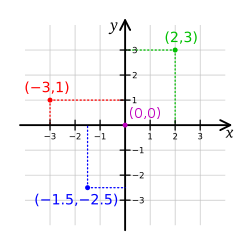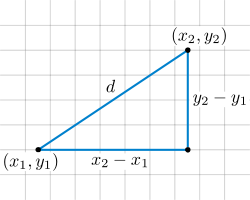Analytic geometry

﻿
Analytic geometryCartesian coordinates.

Analytic geometry, or analytical geometry has two different meanings in mathematics. The modern and advanced meaning refers to the geometry of analytic varieties. This article focuses on the classical and elementary meaning.

In classical mathematics, analytic geometry, also known as coordinate geometry, or Cartesian geometry, is the study of geometry using a coordinate system and the principles of algebra and analysis. This contrasts with the synthetic approach of Euclidean geometry, which treats certain geometric notions as primitive, and uses deductive reasoning based on axioms and theorems to derive truth. Analytic geometry is widely used in physics and engineering, and is the foundation of most modern fields of geometry, including algebraic, differential, discrete, and computational geometry.

Usually the Cartesian coordinate system is applied to manipulate equations for planes, straight lines, and squares, often in two and sometimes in three dimensions of measurement. Geometrically, one studies the Euclidean plane (2 dimensions) and Euclidean space (3 dimensions). As taught in school books, analytic geometry can be explained more simply: it is concerned with defining and representing geometrical shapes in a numerical way and extracting numerical information from shapes' numerical definitions and representations. The numerical output, however, might also be a vector or a shape. That the algebra of the real numbers can be employed to yield results about the linear continuum of geometry relies on the Cantor–Dedekind axiom.

History

The Greek mathematician Menaechmus solved problems and proved theorems by using a method that had a strong resemblance to the use of coordinates and it has sometimes been maintained that he had introduced analytic geometry. Apollonius of Perga, in On Determinate Section, dealt with problems in a manner that may be called an analytic geometry of one dimension; with the question of finding points on a line that were in a ratio to the others. Apollonius in the Conics further developed a method that is so similar to analytic geometry that his work is sometimes thought to have anticipated the work of Descartes — by some 1800 years. His application of reference lines, a diameter and a tangent is essentially no different than our modern use of a coordinate frame, where the distances measured along the diameter from the point of tangency are the abscissas, and the segments parallel to the tangent and intercepted between the axis and the curve are the ordinates. He further developed relations between the abscissas and the corresponding ordinates that are equivalent to rhetorical equations of curves. However, although Apollonius came close to developing analytic geometry, he did not manage to do so since he did not take into account negative magnitudes and in every case the coordinate system was superimposed upon a given curve a posteriori instead of a priori. That is, equations were determined by curves, but curves were not determined by equations. Coordinates, variables, and equations were subsidiary notions applied to a specific geometric situation.

The eleventh century Persian mathematician Omar Khayyám saw a strong relationship between geometry and algebra, and was moving in the right direction when he helped to close the gap between numerical and geometric algebra with his geometric solution of the general cubic equations, but the decisive step came later with Descartes.

Analytic geometry has traditionally been attributed to René Descartes Descartes made significant progress with the methods in an essay entitled Geometry, one of the three accompanying essays published in 1637 together with his Discourse on the Method of Rightly Conducting the Reason in the Search for Truth in the Sciences, commonly referred to as Discourse on Method. This work, written in his native French tongue, and its philosophical principles, provided the foundation for Infinitesimal calculus in Europe.

Abraham de Moivre also pioneered the development of analytic geometry.

Basic principlesIllustration of a Cartesian coordinate plane. Four points are marked and labeled with their coordinates: (2,3) in green, (−3,1) in red, (−1.5,−2.5) in blue, and the origin (0,0) in purple.

Coordinates

In analytic geometry, the plane is given a coordinate system, by which every point has a pair of real number coordinates. The most common coordinate system to use is the Cartesian coordinate system, where each point has an x-coordinate representing its horizontal position, and a y-coordinate representing its vertical position. These are typically written as an ordered pair (xy). This system can also be used for three-dimensional geometry, where every point in Euclidean space is represented by an ordered triple of coordinates (xyz).

Other coordinate systems are possible. On the plane the most common alternative is polar coordinates, where every point is represented by its radius r from the origin and its angle θ. In three dimensions, common alternative coordinate systems include cylindrical coordinates and spherical coordinates.

Equations of curves

In analytic geometry, any equation involving the coordinates specifies a subset of the plane, namely the solution set for the equation. For example, the equation y = x corresponds to the set of all the points on the plane whose x-coordinate and y-coordinate are equal. These points form a line, and y = x is said to be the equation for this line. In general, linear equations involving x and y specify lines, quadratic equations specify conic sections, and more complicated equations describe more complicated figures.

Usually, a single equation corresponds to a curve on the plane. This is not always the case: the trivial equation x = x specifies the entire plane, and the equation x2 + y2 = 0 specifies only the single point (0, 0). In three dimensions, a single equation usually gives a surface, and a curve must be specified as the intersection of two surfaces (see below), or as a system of parametric equations. The equation x2 + y2 = r2 is the equation for any circle with a radius of r.The distance formula on the plane follows from the Pythagorean theorem.

Distance and angle

In analytic geometry, geometric notions such as distance and angle measure are defined using formulas. These definitions are designed to be consistent with the underlying Euclidean geometry. For example, using Cartesian coordinates on the plane, the distance between two points (x1y1) and (x2y2) is defined by the formula$d = \sqrt{(x_2 - x_1)^2 + (y_2 - y_1)^2},\!$

which can be viewed as a version of the Pythagorean theorem. Similarly, the angle that a line makes with the horizontal can be defined by the formula$\theta = \tan^{-1}(m)\!$

where m is the slope of the line.

Section of a Line

In Analytical Geometry a section of a line can be given by the formula where (c,d)&(e,f) are the endpoints of the line & m:n is the ratio of division

S(a,b)=(nc+me/m+n , nd+mf/m+n)

Transformations

Transformations are applied to parent functions to turn it into a new function with similar characteristics. For example, the parent function y=1/x has a horizontal and a vertical asymptote, and occupies the first and third quadrant, and all of its transformed forms have one horizontal and vertical asymptote,and occupies either the 1st and 3rd or 2nd and 4th quadrant. In general, if y = f(x), then it can be transformed into y = af(b(x − k)) + h. In the new transformed function, a is the factor that vertically stretches the function if it is greater than 1 or vertically compresses the function if it is less than 1, and for negative a values, the function is reflected in the x-axis. The b value compresses the graph of the function horizontally if greater than 1 and stretches the function horizontally if less than 1, and like a, reflects the function in the y-axis when it is negative. The k and h values introduce translations, h, vertical, and k horizontal. Positive h and k values mean the function is translated to the positive end of its axis and negative meaning translation towards the negative end.

Themes

Important themes of analytical geometry are

Many of these problems involve linear algebra.

Example

Here an example of a problem from the United States of America Mathematical Talent Search that can be solved via analytic geometry:

Problem: In a convex pentagon ABCDE, the sides have lengths 1, 2, 3, 4, and 5, though not necessarily in that order. Let F, G, H, and I be the midpoints of the sides AB, BC, CD, and DE, respectively. Let X be the midpoint of segment FH, and Y be the midpoint of segment GI. The length of segment XY is an integer. Find all possible values for the length of side AE.

Solution: Without loss of generality, let A, B, C, D, and E be located at A = (0,0), B = (a,0), C = (b,e), D = (c,f), and E = (d,g).

Using the midpoint formula, the points F, G, H, I, X, and Y are located at$F\left(\frac{a}{2},0\right)$,$G\left(\frac{a+b}{2},\frac{e}{2}\right)$,$H\left(\frac{b+c}{2},\frac{e+f}{2}\right)$,$I\left(\frac{c+d}{2},\frac{f+g}{2}\right)$,$X\left(\frac{a+b+c}{4},\frac{e+f}{4}\right)$, and$Y\left(\frac{a+b+c+d}{4},\frac{e+f+g}{4}\right).$

Using the distance formula,$AE=\sqrt{d^2+g^2}$

and$XY=\sqrt{\frac{d^2}{16}+\frac{g^2}{16}}=\frac{\sqrt{d^2+g^2}}{4}.$

Since XY has to be an integer,$AE\equiv 0\pmod{4}$

(see modular arithmetic) so AE = 4.

Modern analytic geometry

An analytic variety is defined locally as the set of common solutions of several equations involving analytic functions. It is analogous to the included concept of real or complex algebraic variety. Any complex manifold is an analytic variety. Since analytic varieties may have singular points, not all analytic varieties are manifolds.

Analytic geometry is essentially equivalent to real and complex Algebraic geometry, as has been shown by Jean-Pierre Serre in his paper GAGA, the name of which is French for Algebraic geometry and analytic geometry. Nevertheless, the two fields remain distinct, as the methods of proof are quite different and algebraic geometry includes also geometry in finite characteristic.

Wikimedia Foundation. 2010.

Look at other dictionaries:

• analytic geometry — n. the branch of geometry in which a coordinate graphing system makes visible, using points, lines, and curves, the numerical relationships of algebraic equations: also called coordinate geometry or Cartesian geometry …   English World dictionary

• analytic geometry — a branch of mathematics in which algebraic procedures are applied to geometry and position is represented analytically by coordinates. Also called coordinate geometry. [1820 30] * * * Investigation of geometric objects using coordinate systems.… …   Universalium

• analytic geometry — noun the use of algebra to study geometric properties; operates on symbols defined in a coordinate system • Syn: ↑analytical geometry, ↑coordinate geometry • Topics: ↑mathematics, ↑math, ↑maths • Hypernyms: ↑ …   Useful english dictionary

• analytic geometry — noun Date: 1835 the study of geometric properties by means of algebraic operations upon symbols defined in terms of a coordinate system called also coordinate geometry …   New Collegiate Dictionary

• analytic geometry — study of geometric properties by means of algebraic operations, coordinate geometry …   English contemporary dictionary

• analytic geometry — analyt′ic geom′etry n. math. a branch of mathematics in which algebraic procedures are applied to geometry and position is represented analytically by coordinates • Etymology: 1820–30 …   From formal English to slang

• analytic geometry — noun a branch of mathematics that investigates properties of figures through the coordinates of their points …   Wiktionary

• Algebraic geometry and analytic geometry — In mathematics, algebraic geometry and analytic geometry are two closely related subjects. While algebraic geometry studies algebraic varieties, analytic geometry deals with complex manifolds and the more general analytic spaces defined locally… …   Wikipedia

• Complex analytic geometry — In mathematics, complex analytic geometry sometimes denotes the application of complex numbers to plane geometry. Rather than represent a point in the plane as a pair of Cartesian coordinates, it can be represented as a single complex number,… …   Wikipedia

• Geometry — (Greek γεωμετρία ; geo = earth, metria = measure) is a part of mathematics concerned with questions of size, shape, and relative position of figures and with properties of space. Geometry is one of the oldest sciences. Initially a body of… …   Wikipedia